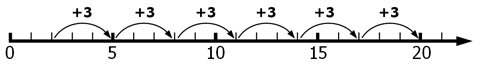Home > Patterns > Good teaching > Concrete to abstract > Making number sequences real

# Making number sequences real

There are many ways in which investigations with objects or shapes lead to number sequences.

• Building a block pattern by repeatedly adding the same unit of repeat leads to a sequence of multiples (see Introducing number sequences).
• Building a staircase leads to a sequence of even numbers (see Making a Staircase).
• Some problem situations lead to interesting number sequences (see Let's have a Party!).
• The number of objects in a growing square array leads to a sequence of square numbers (see Find a rule).
• Hops of a fixed length along a number line give a number sequence linked to addition (or subtraction if the jumps are backwards). For example, starting at 2 and repeatedly adding 3 gives the sequence 2, 5, 8, 11, 14, 17, 20…A number sequence on a number line.

It can be a valuable exercise to reverse this process.

Give students a simple number sequence (one which increases or decreases at a fixed rate) and ask them to come up with a familiar situation which would yield the given sequence.

## A hundred square

A wide variety of number sequences can be found on a hundred square or chart. Exploring them helps students strengthen their knowledge of place value and their addition skills.

## Folding patterns

Number sequences can also be linked to fractions through this folding activity.